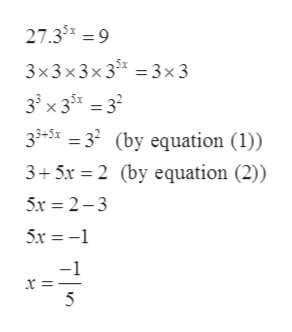Question
2 views

Use like bases to solve the exponential equation.

27 · 35x = 9
check_circle

Step 1

Given:

Step 2

Formula used for solving the given exponential equation is

Step 3

Calculati...help_outlineImage Transcriptionclose27.35* =9 3x3x3x3 3x3 33 x3532 33+5x =32 (by equation (1)) 3+5x 2 (by equation (2)) 5x 2-3 5x -1 5 fullscreen

### Want to see the full answer?

See Solution

#### Want to see this answer and more?

Solutions are written by subject experts who are available 24/7. Questions are typically answered within 1 hour.*

See Solution
*Response times may vary by subject and question.
Tagged in

### Exponents